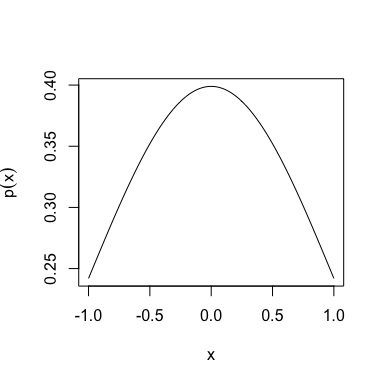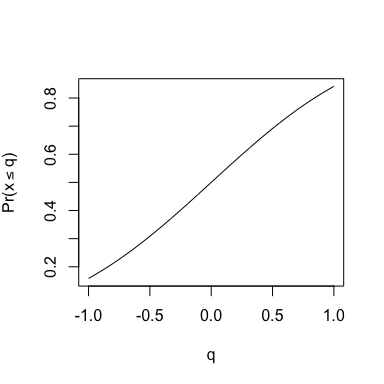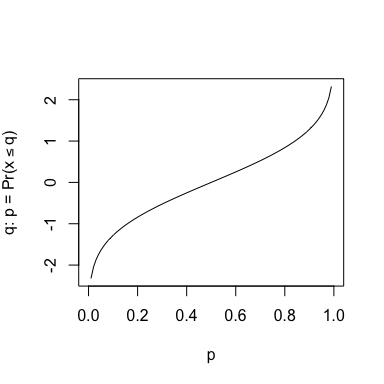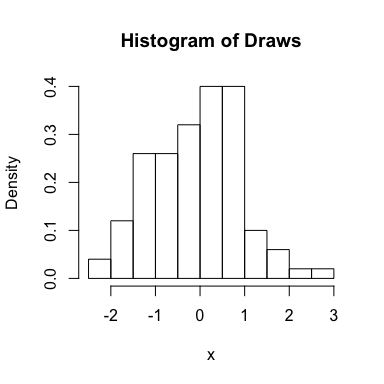# Working with the Exponential Power Distribution Using gnorm

#### 2018-01-29

The exponential power distribution, also known as the generalized normal distribution, was first described in Subbotin (1923)1 and rediscovered as the generalized normal distribution in Nadarajah (2005)2. It generalizes the Laplace, normal and uniform distributions and is pretty easy to work with in many ways, so it can be very useful. Accordingly, I’ve made a little R package called gnorm that provides density, CDF and quantile functions for the exponential power distribution as well as random variate generation that are analogous to dnorm, pnorm, qnorm and rnorm. The package can be installed either via CRAN using install.packages(gnorm) or via Github using the devtools package and the command install_github("maryclare/gnorm"). We can load after installation as follows:

library(gnorm)

Since we’re going to be generating some random variables, we should also set a seed:

set.seed(100)

### Exponential Power Density, dgnorm

An exponential power distributed random variable, $$x$$, has the following density:

$p(x | \mu, \alpha, \beta) = \frac{\beta}{2\alpha \Gamma(1/\beta)}\text{exp}\{-(\frac{|x - \mu|}{\alpha})^\beta\},$ where $$\mu$$ is the mean of $$x$$ and $$\alpha > 0$$ and $$\beta > 0$$ are scale and shape parameters.

The function dgnorm gives the exponential power density given above, $$p(x | \mu, \alpha, \beta)$$, evaluated at specified values of $$x$$, $$\mu$$, $$\alpha$$ and $$\beta$$. Below, we give an example of evaluating the exponential power density using dgnorm for $$\mu = 0$$, $$\alpha = \sqrt{2}$$ and $$\beta = 2$$. Note that this is in fact the standard normal density!

xs <- seq(-1, 1, length.out = 100)
plot(xs, dgnorm(xs, mu = 0, alpha = sqrt(2), beta = 2), type = "l",
xlab = "x", ylab = expression(p(x)))Like dnorm, dgnorm has a log argument, with the default log=FALSE. When log=TRUE is specified, $$\text{log}p(x | \mu, \alpha, \beta)$$ is returned.

#### An Aside on Parametrizing the Exponential Power Distribution

This is not necessarily the most natural way to parametrize the exponential power density. One can replace $$\alpha$$ and $$\beta$$ with the standard deviation of $$x$$, $$\sigma$$, and a shape parameter $$q$$: $p(x | \mu, \sigma, q) = \frac{q}{2\tau}\sqrt{\frac{\Gamma(3/q)}{\Gamma(1/q)^3}}\text{exp}\{-(\frac{\Gamma(3/q)}{\Gamma(1/q)})^{q/2}(\frac{|x - \mu|}{\sigma})^q\}$

This is similar but not identically to the parametrization preferred by Box and Tiao (1973)3. That said, the parametrization using $$\alpha$$ and $$\beta$$ is the one featured on Wikipedia so realistically, it’s the one everyone will find when they look up this distribution, so we’ll use the $$\alpha$$ and $$\beta$$ parametrization for the rest of the vignette.

### Exponential Power CDF, pgnorm

The exponential power CDF is derived as follows: \begin{aligned} \text{Pr}(x \leq q | \mu, \alpha, \beta) &= \int_{-\infty}^q \frac{\beta}{2\alpha \Gamma(1/\beta)}\text{exp}\{-(\frac{|x - \mu|}{\alpha})^\beta\} d x \\ &=\int_{-\infty}^{q - \mu} \frac{\beta}{2\alpha \Gamma(1/\beta)}\text{exp}\{-(\frac{1}{\alpha})^\beta |z|^\beta \} d z \\ &=\Bigg\{\begin{array}{cc} \int_{-\infty}^{q - \mu} \frac{\beta}{2\alpha \Gamma(1/\beta)}\text{exp}\{-(\frac{1}{\alpha})^\beta \left(-z\right)^\beta \} d z & q \leq \mu \\ \int_{-\infty}^{0} \frac{\beta}{2\alpha \Gamma(1/\beta)}\text{exp}\{-(\frac{1}{\alpha})^\beta (-z)^\beta + \int_{0}^{q - \mu} \frac{\beta}{2\alpha \Gamma(1/\beta)}\text{exp}\{-(\frac{1}{\alpha})^\beta z^\beta \} d z & q > \mu \\ \end{array} \\ &=\Bigg\{\begin{array}{cc} \int_{-(q - \mu)}^{\infty} \frac{\beta}{2\alpha \Gamma(1/\beta)}\text{exp}\{-(\frac{1}{\alpha})^\beta z^\beta \} d z & q \leq \mu \\ \int_{0}^{\infty} \frac{\beta}{2\alpha \Gamma(1/\beta)}\text{exp}\{-(\frac{1}{\alpha})^\beta z^\beta dz + \int_{0}^{q - \mu} \frac{\beta}{2\alpha \Gamma(1/\beta)}\text{exp}\{-(\frac{1}{\alpha})^\beta z^\beta \} dz & q > \mu \\ \end{array} \\ &=\Bigg\{\begin{array}{cc} \int_{0}^{\infty} \frac{\beta}{2\alpha \Gamma(1/\beta)}\text{exp}\{-(\frac{1}{\alpha})^\beta z^\beta \} d z - \int_{0}^{-(q - \mu)} \frac{\beta}{2\alpha \Gamma(1/\beta)}\text{exp}\{-(\frac{1}{\alpha})^\beta z^\beta \} d z& q \leq \mu \\ \int_{0}^{\infty} \frac{\beta}{2\alpha \Gamma(1/\beta)}\text{exp}\{-(\frac{1}{\alpha})^\beta z^\beta\} dz+ \int_{0}^{q - \mu} \frac{\beta}{2\alpha \Gamma(1/\beta)}\text{exp}\{-(\frac{1}{\alpha})^\beta z^\beta \} d z & q > \mu \\ \end{array} \\ &= \int_{0}^{\infty} \frac{\beta}{2\alpha \Gamma(1/\beta)}\text{exp}\{-(\frac{1}{\alpha})^\beta z^\beta \}dz+ \text{sign}(q - \mu) \int_{0}^{|q - \mu|} \frac{\beta}{2\alpha \Gamma(1/\beta)}\text{exp}\{-(\frac{1}{\alpha})^\beta z^\beta \} d z \\ &= \int_{0}^{\infty} \frac{w^{1/\beta - 1}}{2\alpha \Gamma(1/\beta)}\text{exp}\{-(\frac{1}{\alpha})^\beta w \}dw+ \text{sign}(q - \mu) \int_{0}^{|q - \mu|^\beta} \frac{w^{1/\beta - 1}}{2\alpha \Gamma(1/\beta)}\text{exp}\{-(\frac{1}{\alpha})^\beta w \} d w && z^\beta = w \\ &= \frac{1}{2}+ \frac{\text{sign}(q - \mu)}{2} \underbrace{\int_{0}^{|q - \mu|^\beta} \frac{w^{1/\beta - 1}}{\alpha \Gamma(1/\beta)}\text{exp}\{-(\frac{1}{\alpha})^\beta w \} d w}_{\text{Gamma CDF, Shape=\frac{1}{\beta}, Rate=(\frac{1}{\alpha})^\beta}} \end{aligned} We can evaluate the exponential power CDF using the gamma CDF - the pgamma function evaluates the CDF of a gamma distribution with parameters $$\frac{1}{\beta}$$ and $$(\frac{1}{\alpha})^\beta$$ at $$|q - \mu|^\beta$$.

The function pgnorm returns the value of the CDF for specified values of $$q$$, $$\mu$$, $$\alpha$$ and $$\beta$$. Like the corresponding pnorm function, it also has the following arguments:

• log.p, which returns the log probability when set to TRUE. The default is log.p=FALSE;
• lower.tail, which returns $$\text{Pr}(x > q | \mu, \alpha, \beta)$$ when set to FALSE. The default is lower.tail=TRUE.

Below, we give an example of evaluating the exponential power CDF using pgnorm for $$\mu = 0$$, $$\alpha = \sqrt{2}$$ and $$\beta = 2$$. As in the previous example - this is the standard normal CDF.

xs <- seq(-1, 1, length.out = 100)
plot(xs, pgnorm(xs, 0, sqrt(2), 2), type = "l", xlab = "q", ylab = expression(paste("Pr(", x<=q, ")", sep = "")))### Exponential Power Quantile Function (Inverse CDF), qgnorm

A (relatively) straightforward way to compute the inverse CDF function for an exponential power random variable is to use its scale-sign representation (Gupta and Varga, 1993)4. We can write $$x \stackrel{d}{=} su + \mu$$, where $$s$$ and $$u$$ are independent random variables with $$p(s | \alpha, \beta) = \frac{\beta}{\alpha \Gamma(1/\beta)}\text{exp}\{-(\frac{s}{\alpha})^\beta\}$$ and $$u$$ uniformly distributed on $$\{-1, 1\}$$.

Given $$p$$, we want to find the value of $$q$$ that satisfies $$p = \text{Pr}(x \leq q | \mu, \alpha, \beta)$$. We can rewrite the problem using $$s$$ and $$u$$ as finding $$p$$ and $$q$$ that satisfy: \begin{aligned} |p - 0.5|&= \text{Pr}(s \leq |q - \mu| | \alpha, \beta)\text{Pr}(u =\text{sign}(q - \mu)) \\ &= \frac{1}{2}\text{Pr}(s \leq |q - \mu| | \alpha, \beta) \\ &= \frac{1}{2}\int_0^{|q - \mu|} \frac{\beta}{\alpha \Gamma(1/\beta)}\text{exp}\{-(\frac{s}{\alpha})^\beta\} ds \\ &= \frac{1}{2}\underbrace{\int_0^{|q - \mu |^\beta} \frac{1}{\alpha \Gamma(1/\beta)}t^{1/\beta - 1}\text{exp}\{-(\frac{1}{\alpha})^\beta t\} dt}_{\text{Gamma CDF, Shape=\frac{1}{\beta}, Rate=(\frac{1}{\alpha})^\beta)}} & s^\beta = t \end{aligned}

Let $$g(2|p - 0.5|; \frac{1}{\beta}, (\frac{1}{\alpha})^\beta)$$ refer to the inverse CDF function for a gamma distribution with shape $$\frac{1}{\beta}$$ and rate $$(\frac{1}{\alpha})^\beta$$ evaluated at $$2|p - 0.5|$$. Then the inverse CDF of the exponential power distribution is given by: $|q - \mu| = g(2|p - 0.5|; \frac{1}{\beta}, (\frac{1}{\alpha})^\beta)^{1/\beta}.$

Noting that $$q > \mu$$ when $$p > 0.5$$ and $$q \leq \mu$$ when $$p \leq 0.5$$, we get: $q = \text{sign}(p - 0.5)g(2|p - 0.5|; \frac{1}{\beta}, (\frac{1}{\alpha})^\beta)^{1/\beta} + \mu.$

This is what is implemented by qgnorm for specified values of $$q$$, $$\mu$$, $$\alpha$$ and $$\beta$$. Like the corresponding qnorm function, it also has the following arguments:

• log.p, which indicates that the log probability has been provided when set to TRUE. The default is log.p=FALSE;
• lower.tail, which indicates that $$q$$ satisfies $$p \text{Pr}(x > q | \mu, \alpha, \beta)$$ when set to FALSE. The default is lower.tail=TRUE.

Below, we give an example of evaluating the exponential power inverse CDF using qgnorm for $$\mu = 0$$, $$\alpha = \sqrt{2}$$ and $$\beta = 2$$. As in the previous examples - this is the standard normal CDF.

xs <- seq(0, 1, length.out = 100)
plot(xs, qgnorm(xs, 0, sqrt(2), 2), type = "l", xlab = "p", ylab = expression(paste("q: p = Pr(", x<=q, ")", sep = "")))### Exponential Power Random Variate Generate, rgnorm

The function rgnorm generates exponential power random variates using the same scale-sign stochastic representation of an exponential power random variable used to compute the inverse CDF. Recall that an exponential power distributed random variable, $$x$$, with parameters $$\mu$$, $$\alpha$$ and $$\beta$$ can be written as $$x \stackrel{d}{=} su + \mu$$, where $$s$$ and $$u$$ are independent random variables with $$p(s | \alpha, \beta) = \frac{\beta}{\alpha \Gamma(1/\beta)}\text{exp}\{-(\frac{s}{\alpha})^\beta\}$$ and $$u$$ uniformly distributed on $$\{-1, 1\}$$

Drawing a value of $$u$$ that is uniformly distributed on $$\{-1, 1\}$$ is simple. We can draw a value $$s$$ according to $$p(s | \alpha, \beta)$$ using the inverse CDF function of $$s$$. For fixed $$p$$, the inverse CDF function of $$s$$ finds the value $$q$$ that satisfies $$p = \text{Pr}(s \leq q | \alpha, \beta)$$:

\begin{aligned} p &= \int_0^q \frac{\beta}{\alpha \Gamma(1/\beta)}\text{exp}\{-(\frac{s}{\alpha})^\beta\}ds \\ &= \int_0^{q^\beta} \frac{1}{\alpha \Gamma(1/\beta)}t^{1/\beta - 1}\text{exp}\{-(\frac{1}{\alpha})^\beta t\}dt & s^\beta = t. \end{aligned} As we’ve seen a few times before, this is a gamma CDF. Let $$g(p; \frac{1}{\beta}, (\frac{1}{\alpha})^\beta)$$ refer to the inverse CDF function for a gamma distribution with shape $$\frac{1}{\beta}$$ and rate $$(\frac{1}{\alpha})^\beta$$ evaluated at $$p$$. Then we can generate $$s$$ as follows by drawing a value of $$p$$ from a uniform distribution on $$(0, 1)$$ and setting $$s = g(p; \frac{1}{\beta}, (\frac{1}{\alpha})^\beta)$$. This is how rgnorm generates draws from the exponential power distribution. The function rgnorm is a parametrized like rnorm. The first argument is an integer n, which gives the number of draws to take, and the second through fourth arguments are values of $$\mu$$, $$\alpha$$ and $$\beta$$.

Below, we give an example of using the rgnorm to draw exponential power random variates for $$\mu = 0$$, $$\alpha = \sqrt{2}$$ and $$\beta = 2$$. As in the previous examples - this is the standard normal CDF.

xs <- rgnorm(100, 0, sqrt(2), 2)
hist(xs, xlab = "x", freq = FALSE, main = "Histogram of Draws")#### An Aside on Alternative Approaches to Exponential Power Random Variate Generation

There at least one other approach to generating exponential power random variables for any value of $$q$$. A uniform scale mixture representation of an exponential power distributed random variable, $$x$$, was originally given in Walker and Guttierez-Pena (1999)5 and was reprinted in Choy and Walker (2002)6. It can be used to generate an exponential power random variate, $$x$$, as follows:

• Draw $$\gamma \sim \text{gamma}(\text{shape} = 1 + 1/\beta, \text{rate}=2^{-\beta/2})$$;
• Set $$\delta = \alpha\gamma^{1/\beta}/\sqrt{2}$$;
• Draw $$x \sim \text{uniform}(\mu -\delta, \mu + \delta)$$.